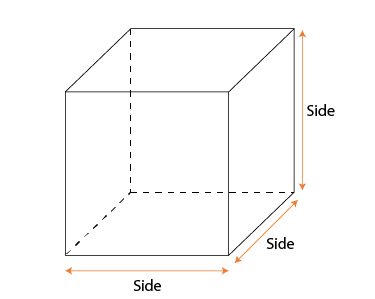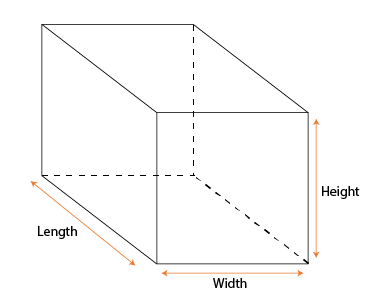Algebraic Identity (a³+b³)

## Objective

To verify the algebraic identity a³ + b³ = (a+b) (a²-ab+b²).

## Theory

### Algebraic Identity

An algebraic identity is an equality, which is true for all values of the variables in the equality.

The algebraic equations which are valid for all values of variables in them are called algebraic identities.

In this way, algebraic identities are used in the computation of algebraic expressions and solving different polynomials

Algebraic identities are also used for the factorization of polynomials.

## Prerequisite Knowledge

### CubeA cube is a three-dimensional solid object bounded by six square faces, facets or sides, with three meeting at each vertex.It has 6 faces, 12 edges, and 8 vertices. for Cube length , width and height are equal.

Volume of Cube

• The product of the length of each side itself.
• Formula : Volume = length * width * height

### CuboidA cuboid is a hexahedron, a six-faced solid. Its faces are quadrilaterals. Cuboid means "like a cube", with 6 squares as faces, a rectangular prism, rectangular cuboid or rectangular box, with 6 rectangles as faces, for both, cube and rectangular prism, adjacent faces meet in a right angle.

#### Volume of Cuboid

• The product of its length and width and height
• Formula : Volume = length * width * height